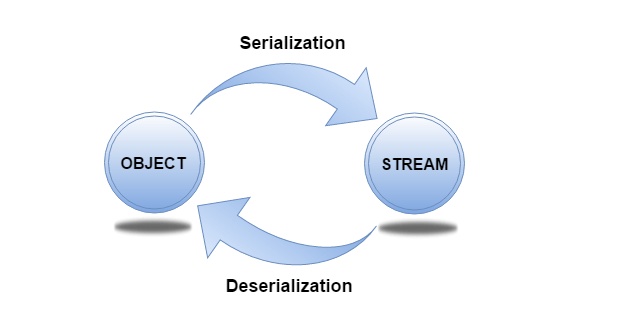# Implementing a Custom Serializer for Yaydoc

At the crux of it, Yaydoc is comprised of a number of specialized bash scripts which perform various tasks such as generating documentation, publishing it to github pages, heroku, etc. These bash scripts also serve as the central communication portal for various technologies used in Yaydoc. The core generator is composed of several Python modules extending the sphinx documentation generator. The web Interface has been built using Node, Express, etc. Yaydoc also contains a Python package dedicated to reading configuration options from a Yaml file.

Till now the options were read and then converted to strings irrespective of the actual data type, based on some simple rules.

• List was converted to a comma separated string.(Nested lists were not handled)
• Boolean values were converted to true | false respectively.
• None was converted to an empty string.

While these simple rules were enough at that time, It was certain that a better solution would be required as the project grew in size. It was also getting tough to maintain because a lot of hard-coding was required when we wanted to convert those strings to python objects. To handle these cases, I decided to create a custom serialization format which would be simple for our use cases and easily parseable from a bash script yet can handle all edge cases. The format is mostly similar to its earlier form apart from lists where it takes heavy inspiration from the python language itself.

With the new implementation, Lists would get converted to comma separated strings enclosed by square brackets. This allowed us to encode the type of the object in the string so that it can later be decoded. This handled the case of an empty list or a list with single element well. The implementation also handled nested lists.

Two methods were created namely serialize and deserialize which detected the type of the corresponding object using several heuristics and applied the proper serialization or deserialization rule.

```def serialize(value):
"""
Serializes a python object to a string.
None is serialized to an empty string.
bool values are converted to strings True False.
list or tuples are recursively handled and are comma separated.
"""
if value is None:
return ''
if isinstance(value, str):
return value
if isinstance(value, bool):
return "true" if value else "false"
if isinstance(value, (list, tuple)):
return '[' + ','.join(serialize(_) for _ in value) + ']'
return str(value)
```

To deserialize we also had to handle the case of nested lists. The following snippet does that properly.

```def deserialize(value, numeric=True):
"""
Deserializes a string to a python object.
Strings True False are converted to bools.
`numeric` controls whether strings should be converted to
ints or floats if possible. List strings are handled recursively.
"""
if value.lower() in ("true", "false"):
return value.lower() == "true"
if numeric and _is_numeric(value):
return _to_numeric(value)
if value.startswith('[') and value.endswith(']'):
split = []
element = ''
level = 0
for c in value:
if c == '[':
level += 1
if level != 1:
element += c
elif c == ']':
if level != 1:
element += c
level -= 1
elif c == ',' and level == 1:
split.append(element)
element = ''
else:
element += c
if split or element:
split.append(element)
return [deserialize(_, numeric) for _ in split]
return value
```

With this new approach, we are able to handle much more cases as compared to the previous implementation and is much more robust. It does however still lacks lacks certain features such as serializing dictionaries. That may be be implemented in the future if need be.